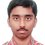# Number Theory problem

For how many positive integral values of N is the expression N(N-101) the perfect square of a positive integer ?

I got 2601 as the only value. I want to know if there are other such values ? I know it's a silly question for Brilliant problem solvers. Please help.........Note by Nishant Sharma
5 years, 10 months ago

MarkdownAppears as
*italics* or _italics_ italics
**bold** or __bold__ bold
- bulleted- list
• bulleted
• list
1. numbered2. list
1. numbered
2. list
Note: you must add a full line of space before and after lists for them to show up correctly
paragraph 1paragraph 2

paragraph 1

paragraph 2

[example link](https://brilliant.org)example link
> This is a quote
This is a quote
    # I indented these lines
# 4 spaces, and now they show
# up as a code block.

print "hello world"
# I indented these lines
# 4 spaces, and now they show
# up as a code block.

print "hello world"
MathAppears as
Remember to wrap math in $$...$$ or $...$ to ensure proper formatting.
2 \times 3 $$2 \times 3$$
2^{34} $$2^{34}$$
a_{i-1} $$a_{i-1}$$
\frac{2}{3} $$\frac{2}{3}$$
\sqrt{2} $$\sqrt{2}$$
\sum_{i=1}^3 $$\sum_{i=1}^3$$
\sin \theta $$\sin \theta$$
\boxed{123} $$\boxed{123}$$

Sort by:

You are missing another obvious (positive integral) solution. (This statement is false. See edit below.)

Hint: Greatest Common Divisor.

Edit: I missed out that $$N (N-101)$$ has to be the square of a positive integer. I was thinking that $$N=101$$ works.

Staff - 5 years, 10 months ago

Seriously could not follow you. Please extrapolate a bit.

- 5 years, 10 months ago

I don't think that any other solution exists, Calvin Sir. Both $$N$$ and $$N(N-101)$$ are to be positive.

- 5 years, 9 months ago

yes

- 5 years, 9 months ago

Me too. That was why I posted this since I had done few problems only. BTW did you also write ISI 2013 ?

- 5 years, 9 months ago

I misread, didn't see "positive" in the latter instance. Thanks for correcting.

Staff - 5 years, 9 months ago

it came in isi right

- 5 years, 10 months ago

yea,you too gave isi,how many could you answer in part i & II...?

- 5 years, 10 months ago

Yeah it was an ISI problem. Well I attempted 29/30 in part I and solved 4 in part II. How about you both ?

- 5 years, 10 months ago

i am 13 so i cannot participate but i saw the question and tried to solve it . i got about 28 in part 1 and 7 in part 2

- 5 years, 10 months ago

Are you sure or just kidding(your age) ? Anyway, where from did you get the questions ?

- 5 years, 10 months ago

y kiddin and q from http://cheentaganitkendra.blogspot.in/2013/05/isi-2013-bmath-bstat-subjective-paper.html

- 5 years, 10 months ago

Let $$x^2=N$$ and

$$y^2=N-101$$ where $$x>0,y>0$$

Now we can write from our first statement that :

$$y^2=x^2-101$$

$$\Rightarrow x^2-y^2=101$$

$$(x+y)(x-y)=101$$

Therefore it follows that $$(x+y)$$ and $$(x-y)$$ are factors of $$101$$.

Now since $$101$$ is a prime and $$x,y$$ are positive integers then the only possible values that $$(x+y)$$ can take is $$101$$.

Note that since $$x>y$$ $$,$$ $$(x-y) \neq 101$$.

So the only solution possible is when $$x=51$$ which gives $$y=50$$.

Therefore $$N=x^2=2601$$ is the only solution.

- 5 years, 9 months ago

You need to substantiate your first line. Why must we have $$N=x^2$$? You are merely given that $$N$$ is an integer, and not told that it is a square. For example, if we ignore the condition that $$N(N-101)$$ must be positive, then $$N=101$$ will be a solution, but that disagrees with your claim that $$N$$ must be a perfect square.

Staff - 5 years, 9 months ago

If $$N=101$$ is a solution then $$y=0$$ but I already stated that $$y>0$$. So N=101 cannot be a solution.

- 5 years, 9 months ago